###### Research Article

Submitted: 03 September 2019 | Approved: 19 September 2019 | Published: 20 September 2019

How to cite this article: Kaganov VI. Arrhythmia of the heart - computer analysis. J Cardiol Cardiovasc Med. 2019; 4: 131-134.

Keywords: Arrhythmia; Heart model; Synchronization; Computer analysis# Arrhythmia of the heart - computer analysis

#### VI Kaganov*

MIREA, Russian Technological University, Russia

*Address for Correspondence: VI Kaganov, MIREA, Russian Technological University, Russia, Tel: +7 9175097166; Email: Kaganovwil@yandex.ru; Kaganv@mirea.ru

###### Abstract

The problem of synchronization of oscillations of various physical nature is discussed. From the standpoint of the theory of synchronism, a model of the heart is considered as a system of four connected between self-oscillating links: two atria and two ventricles. The synchronous and asynchronous operating modes are considered at sinusoidal and relaxation oscillations. A computer program has been compiled that simulates the fluctuations in the heart using four differential equations. Four examples of calculation according to the program are given for asynchronous and synchronous operation modes. The possibility of evaluating the ablation procedure from the perspective of a computer model is discussed.

###### Annotation

According to who terminology cardiac arrhythmia is a pathological human condition associated with a violation of rhythmic and consistent excitation and contraction of the heart compared to normal sinus rhythm.

A patient with arrhythmia feel the accelerated or on the contrary slow heartbeat and sudden loss of strength. Medical diagnosis of arrhythmia is carried out using several methods, including electrocardiograms (ECG), showing the intensity of the electric field of low intensity between different parts of the patient’s body. Thus, with the help of an ECG, the diagnosis of arrhythmia is carried out not by direct, direct examination of the heart, but by secondary signs.

Four ECGs, the first of which reflects the normal, rhythmic functioning of the heart (60-100 beats per minute), and the other three - arrhythmia, are pre-sented in figure 1a,b [1,2]. The second ECG (rts.1, b) reflects sinus bradyordia, in which the frequency of heart beats decreases to 30-50 beats per minute; the third (Figure 1c) - sinus tachycardia associated with their acceleration up to 100 beats per minute; the fourth (Figure 1d) - atrial fibrillation, associated with the appearance of additional small impulses.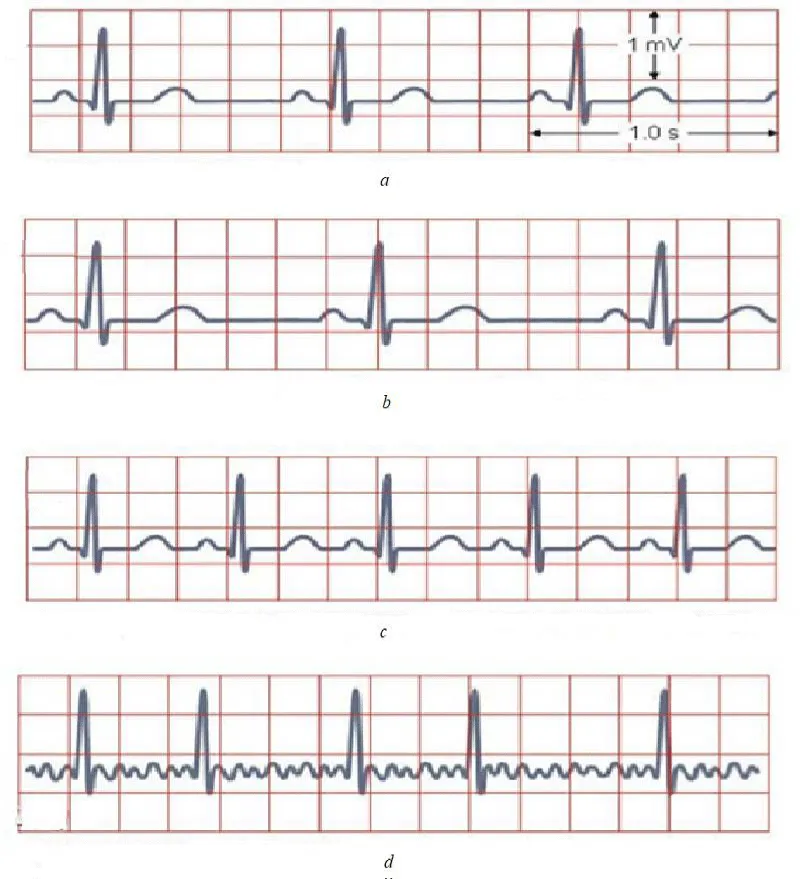Figure 1: Four ECGs, the first of which reflects the normal, rhythmic functioning of the heart (60-100 beats per minute), and the other three - arrhythmia, are presented.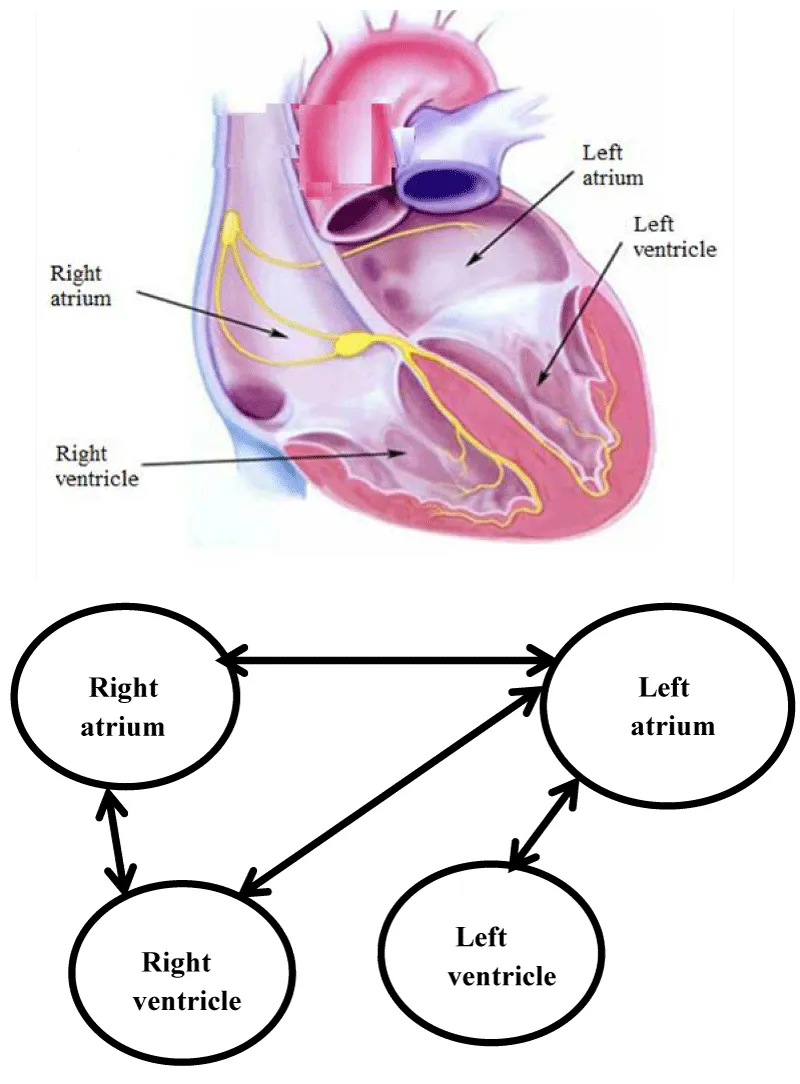Figure 2: The human heart also obeys the synchronization law, in which two atria (right and left) and two ventricles.

A detailed description of different types of ECG and diagnosis of heart disease are given in many works, including [2,3]. Despite the importance and significance of such studies, a natural desire arises in a direct analysis of the work of the heart, and not by secondary characteristics - the analysis of electric fields in the human body, presented in the form of an ECG. Such a search can be carried out using computer analysis, based on the theory of synchronism .

In structures of various physical nature (mechanical electronic, biological, and other types), the existence of a synchronism regime is possible, i.e. the exact coincidence of fluctuations in the shape and frequency of the set of weakly interconnected identical objects.

So, for example, the pendulums of a group of watches placed on a common wall made of a material transmitting vibrations begin to oscillate absolutely precisely. This phenomenon, called synchronization of vibrations, was first discovered by the Dutch physicist Christian Huygens in the middle of the seventeenth century.

So, synchronization is a physical phenomenon, consisting in the exact coincidence of the vibrations of a group of interconnected objects. The human heart also obeys the synchronization law, in which two atria (right and left) and two ventricles (Figure 2a) [4,5], connected between themselves, are constantly and continuously reduced in a single rhythm.

Violation of the synchronous oscillation of these links leads to many internal serious human diseases, united by the common name cardiac arrhythmia [5,6].

Without offering new recommendations for the fight against cardiac arrhythmia, we will consider this phenomenon from the standpoint of mathematics, which will probably allow us to more deeply comprehend the existing methods of treating this human disease.

The basis of mathematical analysis is the following:

- Four organs of the heart that perform rhythmic vibrations are represented as a combination of four self-oscillating links (Figure 2b),

- Connected between these links, carried out by the conductive layer of the myocardium and vessels, is estimated using some coefficients,

- The whole model is described using the following four differential equations (1).

where a1 - a4, b1 - b4 parameters that affect the amplitude and time of establishment of self-oscillations, k1 - k126 are the coupling coefficients between the links;

τ = ωt is the oscillation phase, ω = 2π/t = 2π f is the circular frequency of the self-oscillations of the link, T is the period of these oscillations in seconds, f = 1/T is the oscillation frequency in hertz;

q1 - q4 are the coefficients that determine the ratio of the frequency of natural vibrations of each link to the frequency of synchronous vibrations,

µ1– µ4 are parameters that determine the shape of the vibrations (for µ <1, the vibrations are close to sinusoidal, and for µ> 3 to relaxation).

Note that in a healthy person and f 0 = 1 - 1.66 Hz, which corresponds to 60-100 beats per minute. Outside the zone of the indicated values, arrhythmia may occur. In the examples considered below, the following parameter values are taken as the basis of the normal heart function: T0 = 1s and f 0 = 1 Hz.

We present the results of the calculation according to the program with several characteristic examples related to cardiac arrhythmias. In all examples, the functions U1 (x), U2 (x), U3 (x), U4 (x) determine the vibrations of each of the links heart, and the function UВ (x) is the sum of the four given oscillations.

##### Example 1

One of the self-oscillating links of the heart falls out of synchronism due to the equality to zero, the related coupling coefficients k I = 0. Moreover, the frequency of natural vibrations of such a link is significantly different from the other three. In view of the above, we take: q1 = 1.2; q2 = 1.2; q3 = 1.2 and q4 = 10 (for the defective link). The calculation results for the program of graphs are shown in figure 3.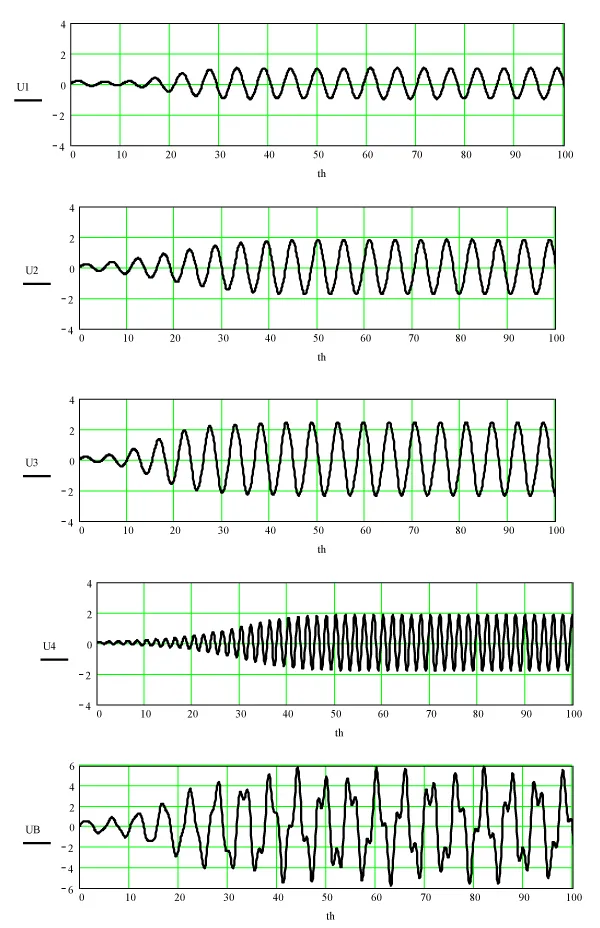Figure 3: The calculation results for the program of graphs.

##### Example 2

Two links and four drop out of synchronism mode. The natural frequency of these links is significantly different from the other two x. In view of the above, we accept:

q1 = 1.2; q2 = 1.2 and q3 = 6; q4 = 10 (defective links). The calculation results for the program of graphs are shown in figure 4.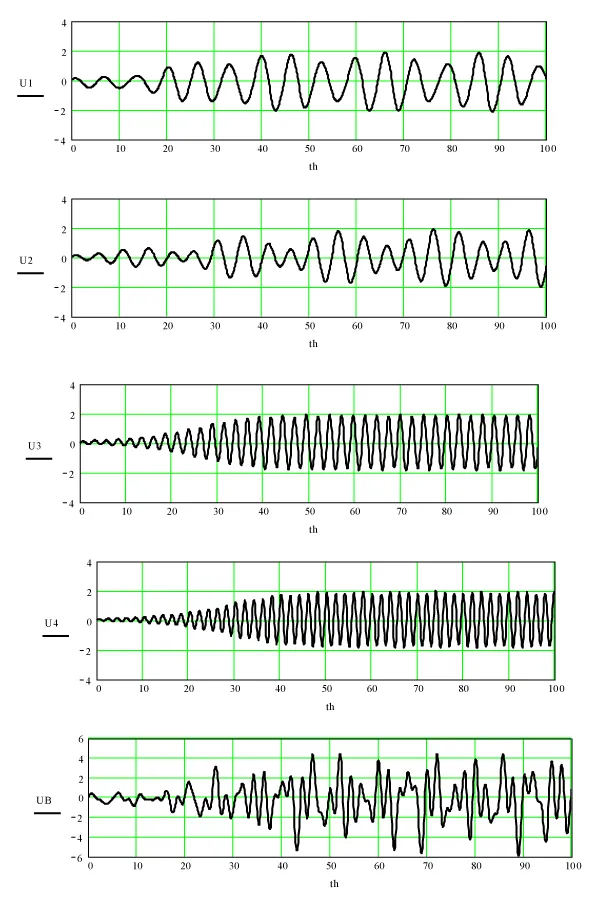Figure 4: Two links and four drop out of synchronism mode.

##### Example 3

All links and connections between them have no defects, as a result of which a normal synchronism mode is established in the heart, which means fluctuations of all links in a single rhythm and normal heart function. An example of such identical oscillations and their sums is shown in figure 5.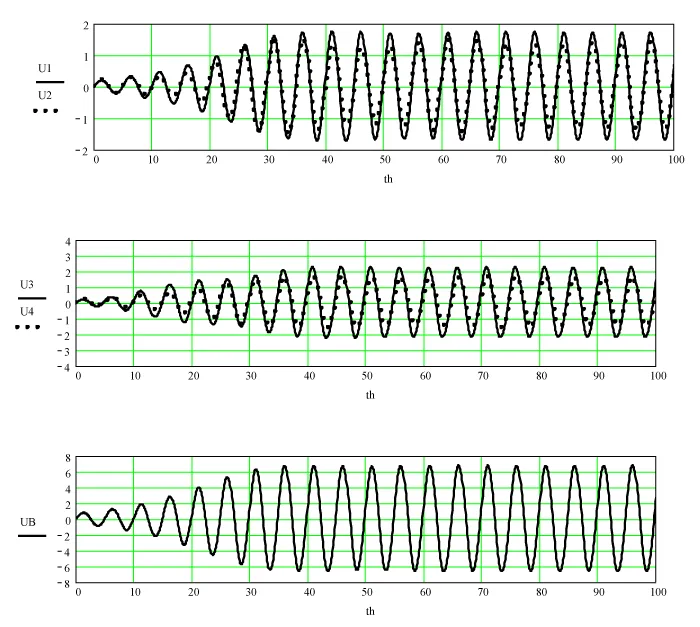Figure 5: Identical oscillations and their sums.

##### Example 4

Oscillations of all links are different from sinusoidal and are relaxing in nature. The values of the parameters µi > 3 correspond to this case. Graphs of the oscillations of the links and their sum at µi = 10 are shown in figure 6.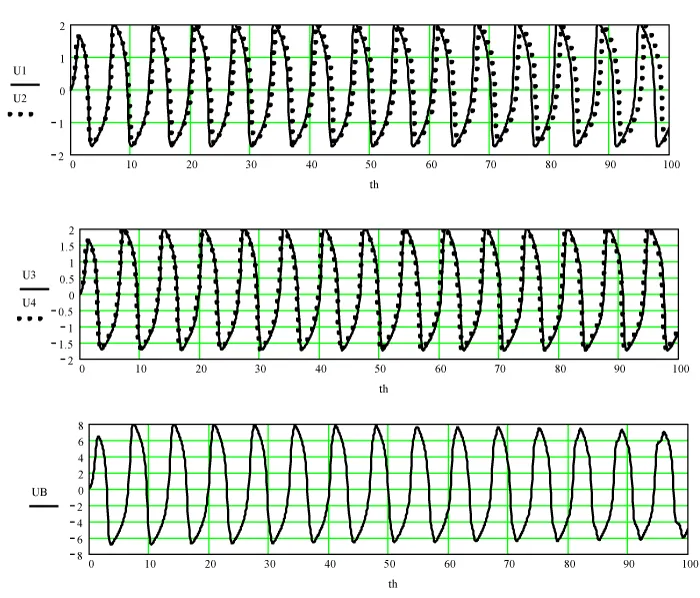Figure 6: Graphs of the oscillations of the links and their sum at µi = 10.

Note that on all the graphs along the abscissa axis the phase of oscillations x = ωt is plotted, where the circular frequency ω = 2π/T, T is the period of these oscillations. A healthy heart has T = 0.7 - 1 s, which corresponds to 60-90 beats per minute. According to the given relation in the constructed graphs, knowing the period of oscillations T, it is easy to switch from phase to time.

We give one of the possible interpretations of the considered examples of computer analysis. Referring to the procedure “ablation” . As you know, ablation consists in exposure to tissue proteins and rejection of part of the cells in the structure of the heart through radio-frequency or laser radiation. From the perspective of the model under consideration, this procedure can be interpreted as the establishment of the required coupling coefficients ki between the four vibrating links of the heart to ensure a stable mode of synchronism in its work.

###### Conclusion

1. The compiled computer model in the form of a system of four differential equations does not open up new methods for treating arrhythmia, but only helps from a mathematical point of view to more deeply comprehend the disturbances in the rhythmic, synchronous work of the heart and in ways to over-come this ailment.

2. According to the calculations and the graphs built on their basis, it follows that with the synchronous operation of the heart, the total oscillation is “smooth”, sinusoidal. Asynchronous operation of the heart (the first sign of arrhythmia), characterized by a distorted form of the total oscillation, can serve as an indicator of a violation of a particular part of the heart.

3. Using the technique of ejaculation of the heart and developing a device for converting the captured sound signals of the heart into electrical vibrations, it will be possible to experimentally verify the results obtained in the frame-work of the developed computer model of the heart.

###### References
1. Ebert G. Simple ECG analysis. Logosphere. 2010.
2. Arrhythmia of the heart. Causes of cardiac arrhythmias, symptoms and treatment.
3. Kaganov VI. Oscillations and waves in nature and technology. M Hotline-Telecom. 2008.
4. Anatomy and physiology of the cardiac conduction system. Ed AV Ardasheva. 2009.
5. Kositsky GI. Human physiology. M Medicine. 1985.\
6. Heart ablation is one of the methods for treating arrhythmia. The Internet.